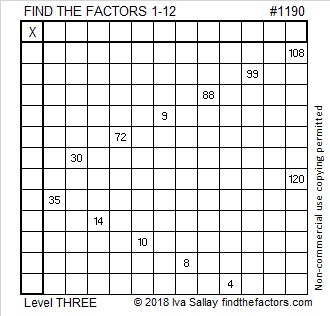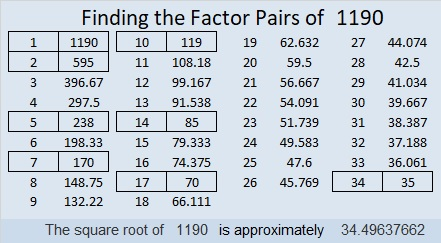# 1190 and Level 3

The common factors of 108 and 120 are 1, 2, 3, 4, 6, and 12, but pick the one that will only put numbers from 1 to 12 in the first column. Then work down that first column cell by cell writing in the factors of the clues as you go. Each number from 1 to 12 must go somewhere in both the first column and the top row.Print the puzzles or type the solution in this excel file: 12 factors 1187-1198

Now I’ll share some information about the number 1190:

• 1190 is a composite number.
• Prime factorization: 1190 = 2 × 5 × 7 × 17
• The exponents in the prime factorization are 1, 1, 1, and 1. Adding one to each and multiplying we get (1 + 1)(1 + 1)(1 + 1)(1 + 1) = 2 × 2 × 2 × 2 = 110. Therefore 1190 has exactly 110 factors.
• Factors of 1190: 1, 2, 5, 7, 10, 14, 17, 34, 35, 70, 85, 119, 170, 238, 595, 1190
• Factor pairs: 1190 = 1 × 1190, 2 × 595, 5 × 238, 7 × 170, 10 × 119, 14 × 85, 17 × 70, or 34 × 35
• 1190 has no square factors that allow its square root to be simplified. √1190 ≈ 34.49638Because 1190 is the product of consecutive numbers 34 and 35, we know it is the sum of the first 34 EVEN numbers. Instead of writing all of those 34 numbers, we can use a some mathematical shorthand and simply write:
2 + 4 + 6 + 8 + . . . + 64 + 66 + 68 = 1190

1190 is the hypotenuse of FOUR Pythagorean triples:
182-1176-1190 which is 14 times (13-84-85)
504-1078-1190 which is 14 times (36-77-85)
560-1050-1190 which is (8-15-17) times 70
714-952-1190 which is (3-4-5) times 238

I like the way 1190 looks in some other bases:
It’s 707 in BASE 13 because 7(13²) + 7(1) = 7(169 + 1) = 7(170) = 1190,
545 in BASE 15,
2A2 in BASE 22,
1C1 in BASE 29 (C is 12 base 10),
and Y0 in BASE 35 (Y is 34 base 10) because 34(35) = 1190

This site uses Akismet to reduce spam. Learn how your comment data is processed.